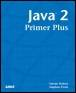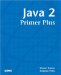# ConstantsJava™ 2 Primer PlusBy Steven Haines, Steve Potts Table of Contents Chapter 2.  Keywords, Data Types, and Variables

 Now that you have data types that represent memory in a specific way and variables as a convention to refer to that memory, how would you represent values that do not change, for example pi? You could define a variable named pi as follows: ` double pi = 3.14285 ` When you need to use it, you could simply reference it in an equation: ` double r = 10; double area = pi * r * r; ` Variables can, as their name implies, contain variable data. But because variables such as pi really cannot change, you must prevent the user from doing the following: ` pi = pi / 2; ` This is perfectly legal in Java, but violates the property of pi. The answer is that we can declare a variable to be a constant, or not changing. Java uses the keyword final to denote that a variable is in fact a constant. Now you can preface your declaration of pi with the keyword final and ensure that its value cannot change: ` final double pi = 3.14285 ` When you try to compile a statement that modifies a constant value, such as ` final double pi = 3.14285 pi = pi / 2; ` The compiler generates an error saying that you cannot assign a value to a final variable. By convention, programmers usually capitalize all the letters in a constant's name so that it can be found with only a casual glance of the source code. Pi, therefore, would be declared as follows: ` final double PI = 3.14285; `TopJava 2 Primer Plus
ISBN: 0672324156
EAN: 2147483647
Year: 2001
Pages: 332

Similar book on Amazon